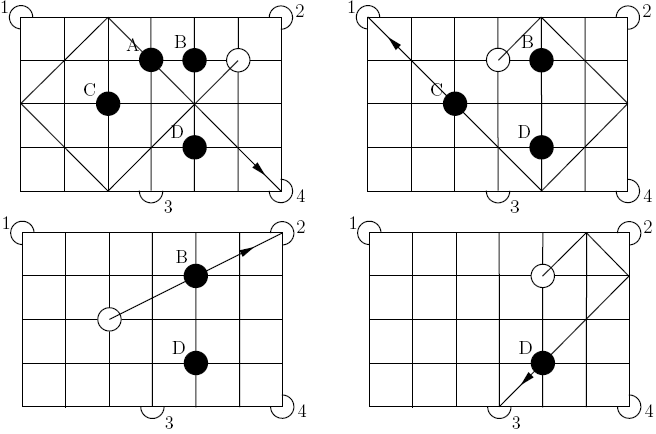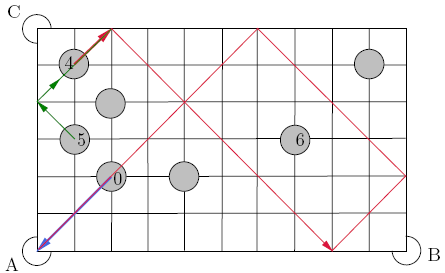MATH EXPLORERS' CLUB Cornell Department of Mathematics

# Billiards and Puzzles

 Introduction1. Rectangular Billiards 2. Circular/Elliptical Tables 3. Triangular Billiards 4. Puzzles 5. Two-Hole Billiards References## Puzzles

Puzzle #1: Consider a rectangular billiard table with 4 pockets, as shown in the figure. One has to put four black balls into four different pockets one at a time, by hitting the white ball. As usual, the balls must bounce from the border with mirror symmetry as we have seen so far. When the white ball hits a black ball, it replaces that black ball's location, and the black ball continues the natural motion. In this process, black balls must never hit each other. This puzzle was used in the PQSRT puzzle competition, Reference #3.The solution is rather simple. However we have to remember that the white ball will always replace the black ball that it hits. Therefore we cannot simply hit ball D and hope it will enter in the third pocket, because then the white ball will bounce from hitting D and will not take D's place. So the first attempt is to actually hit the border at an angle of 45 degrees so that the bouncing is easier. In this way one follows more easily the trajectory of the white ball. For example, a possible sequence of hitts is ball A in the fourth pocket, ball C in the first, ball B in the second, and ball D in the third pocket. The solution is depicted below.Exercise #1: Is it possible to solve the puzzle by putting first C in pocket #1, then D in pocket #4. In what pocket will then ball B go?
Exercise #2: Assume that we can place at most two black balls in the same pocket. Solve the puzzle, but without pocket #3. Then do the same if pocket #2 is missing.

We now look at a rectangular billiard that does not have a special white ball with which to hit the other balls. One simply hit the balls directly. In principle this should make the puzzle less complicated, but as we will see it is not so.

Puzzle #2: Consider the following billiard table with pockets only at three corners and with 7 light grey balls placed as in the picture. One has to put the balls one by one in any pocket so that each ball touches the border a different amount of time, from 0 to 6 exactly once. Moreover, every movement is on 45 diagonals. Fill respective digits into the balls and draw the corresponding paths. This puzzle (slightly changed) was used for the French World Puzzle Championship qualifying test, Reference #6.It is straightforward to find a ball that has zero touches with the border of the table. For example, we choose the ball that is closest to pocket A and denote that 0. The problem becomes more complicated from this point on. It is quite hard to find the ball that has exactlt one touch with the border. As a further hint on how to solve the problem completely, ball #1 will be the next ball to find after 0, 4, 5, and 6 have been found.

Remark: Because every movement is on 45 diagonals, one should avoid hitting the other balls. Hence only bounces from the cushion are allowed.
After a few attempts we decide to start with the ball closest to C and describe its trajectory in red on the picture below. This ball will be 4 and will also enter to pocket A. Ball number 5 is then easier to draw because it will simply follow 4's trajectory with one additional boundary touch, depicted in green. As a further hint, ball number 6 will also follow 5's and then 4's trajectory and enter in pocket A. After that, the puzzle becomes easier since the maximum number of bounces is 3.Exercise #3 Draw the path for ball 6. Continue the solution and write down numbers 1, 3, and 2 on the corresponding balls.

 Top Next lesson